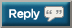# Thread: If you're right, you get repped. If you're wrong, you get negged. (semi-srs)

1. ## If you're right, you get repped. If you're wrong, you get negged. (semi-srs)

1 = 5
2 = 10
3 = 15
4 = 20
5 = ?2. 3503. 69, coz thats the only number that matters to me4. Question wasn't how many cock's have you had inside you.5. 5 = 1 ? I'm thinking this is a trick question somehow.6. 25?7. 1..8. it equals 309. math city bitch math math city bitch 10 10 10 20 is 350 bitch10. Potato11. 1=5
5=1
OP=Phaggot12. 5=113. 1=5
5=1

So 35014. 1 hehe15. 5=116. Answer is 1. It's counter intuitive because one would want to follow the apparent pattern, however since in the first part he said 1 = 5, he already assigned the value of 5 to 1, so 5 = 1.17. Question wasn't how many cock's have you had inside you.
haha someone rep this man18. ran out of reps, gj to the ppl who answered 1..

and to the people who answered 25..19. Answer is 1. It's counter intuitive because one would want to follow the apparent pattern, however since in the first part he said 1 = 5, he already assigned the value of 5 to 1, so 5 = 1.
Mirin Repped20. 1 = 5 therefore 5 = 121. Answer is 1. It's counter intuitive because one would want to follow the apparent pattern, however since in the first part he said 1 = 5, he already assigned the value of 5 to 1, so 5 = 1.
You being a computer science brah should know that if he were checking for equality it would be '==' . Answer could be anything.22.23. one24. its 125. 5=1 cuz 1=526. Does that mean .999 is 1 since 1/3 = .333?27. 5=528. Seen this before. 1.29. 1 = 5
2 = 10
3 = 15
4 = 20
5 = ?